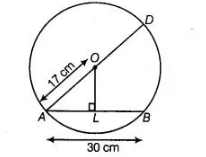# AD is a diameter of a circle and AB is a chord.`
Question:

AD is a diameter of a circle and AB is a chord. If AD = 34 cm, AB = 30 cm, the distance of AB from the centre of the circle is

(a) 17 cm

(b) 15 cm

(c) 4 cm

(d) 8 cm

Solution:

(d) Given, AD = 34 cm and AB = 30 cm

In figure, draw OL ⊥ AB.

Since, the perpendicular from the centre of a circle to a chord bisects the chord.$\therefore$ $A L=L B=\frac{1}{2} A B=15 \mathrm{~cm}$

In right angled $\triangle O L A, \quad O A^{2}=O L^{2}+A L^{2}$ [by Pythagoras theorem]

$\therefore$ $(17)^{2}=O L^{2}+(15)^{2}$

$\Rightarrow$ $289=O L^{2}+225$

$\Rightarrow \quad O L^{2}=289-225=64$

$\therefore \quad O L=8 \mathrm{~cm}$

[taking positive square root, because length is always positive]

Hence, the distance of the chord from the centre is $8 \mathrm{~cm}$.